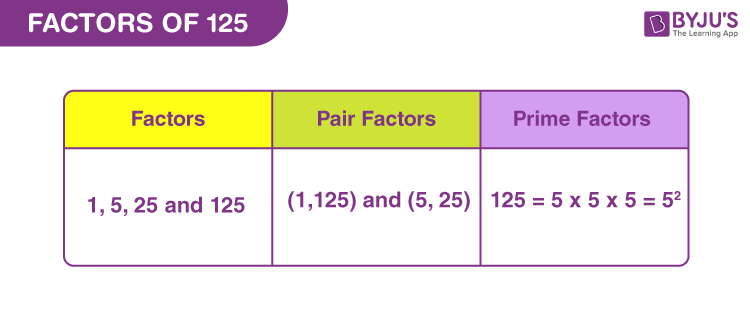Factors of 125 are those whole numbers that divide the original number, evenly. It means that there is no remainder left after dividing 125 by its factor. Therefore, the factor of any number is the proper divisor of the actual number. For example, 5 is the factor of 125 because when we divide 125 by 5 we get;

125 divided by 5 ⇒ 125 ÷ 5 = 25

Thus, we get a whole number as the quotient after division.

In the same way, we can find the other factors of 125 here in this article. Also, we will come across the pair factors of 125. These pair factors result in original numbers upon multiplication with each other. Prime factors of a 125 are the primes that can evenly divide the original number.## How to Find Factors of 125?

Factors of 125 are the numbers that divide the original number, without leaving any remainder. We will start dividing 125 by the smallest natural number 1. As we know, 125 is an odd number, therefore, it cannot be divided by any even number.

125 ÷ 1 = 125

125 ÷ 5 = 25

125 ÷ 25 = 5

125 ÷ 125 = 1

If we divide 125 by any other number between 1 and 125, then the quotient will be a fraction and not a whole number. For example, 125 divided by 10 is 12.5.

Thus, we can conclude that only 1, 5, 25 and 125 are the factors of 125.

## Pair Factors of 125

To find the pair factors of 125, we need to find the product of the two numbers, such that we get the original number as 125.

1 × 125 = 125

5 × 25 = 125

Therefore, the pair factors are (1, 125) and (5, 25). These are the positive pair factors.

Similarly, if we consider negative pair factors then the multiplication of two negative factors will result in a positive value.

-1 × -125 = 125

-5 × -25 = 125

Therefore, the negative pair factors are (-1, -125) and (-5, -25).

## Prime Factorisation of 125

To find the prime factors of 125 we need to divide it by prime numbers till the remainder is 1.

Step 1: Dividing 125 by the smallest prime factor, which is 5, we get;

125/5 = 25

Step 2: Again divide 25 by the smallest prime factor, 5, to get;

5/5 = 1

Step 3: We cannot divide 1 any further by prime factors. Therefore,

 Prime factorisation of 125 = 5 x 5 x 5

## Video Lesson on Prime Factors## Solved Examples

Q.1: If there are 125 books to be distributed among 25 students in a class. How many books does each student get?

Solution: Given,

Number of books = 125

Number of students in class = 25

Each student will get = 125/25 = 5 books

Q.2: What is the sum of all the factors of 125?

Solution: The factors of 125 are 1, 5, 25 and 125.

Sum = 1+5+25+125 = 156

Therefore, 156 is the required sum.

Q.3: What are the common factors of 25 and 125?

Answer: To find the common factors, we need to write down the factors of each of the given numbers.

Since, 25 and 125 are both composite numbers, thus, they will have more than two factors.

25→ 1,5 and 25

125 → 1,5,25 and 125.

We can see, the common factors are 1, 5 and 25.

## Frequently Asked Questions on Factors of 125

### How many factors of 125 are there?

There are four factors of 125. They are 1, 5, 25 and 125.

### Is 125 also a multiple of itself?

125 is both factor and multiple of 125. 125 divided by 125 is equal to 1 and inversely, 125 multiplied by 1 is equal to 125.
125 ÷ 125 = 1 (125 is Factor)
125 x 1 = 125 (125 is multiple)

### What are the multiples of 125?

The first 10 multiples of 125 are 125, 250, 375, 500, 625, 750, 875, 1000, 1125 and 1250.

### What are the prime factors of 125?

The prime factorisation of 125 is equal 5 x 5 x 5 = 53. Therefore, 5 is the prime factor of 125.

### What is the average of factors of 125?

Average of factors is the sum of all the factors divided by total number of factors.
Average (factors of 125) = (1+5+25+125)/4 = 156/4 = 39
Therefore, the required average is 39.